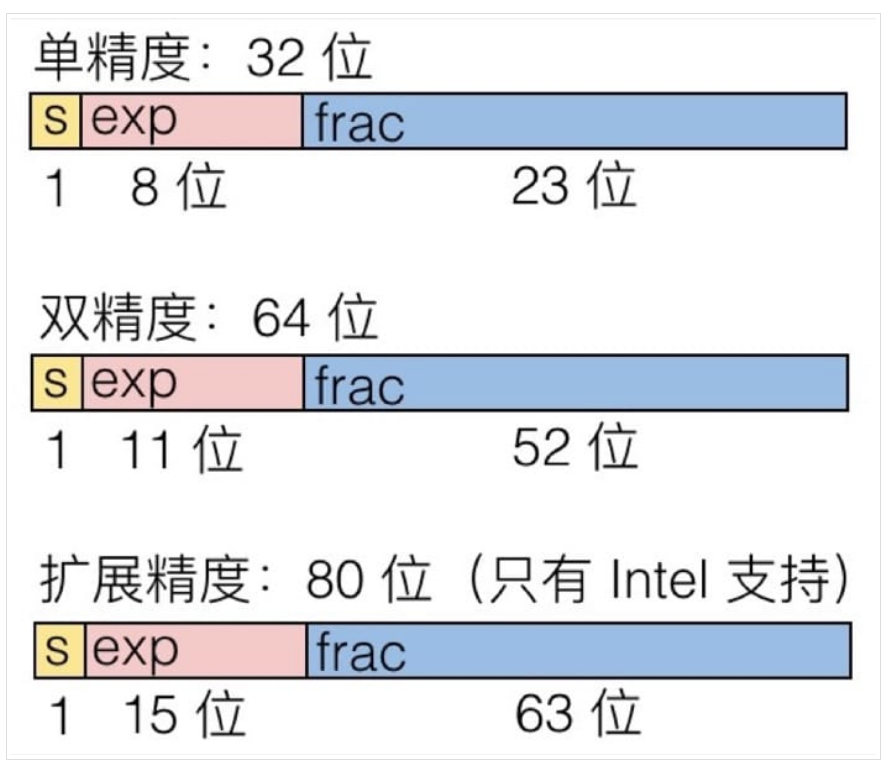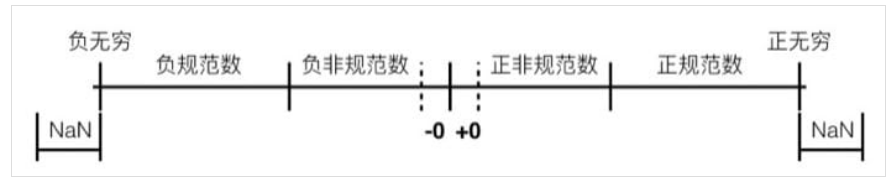# IEEE 754表示浮点数• 规格化的，exp部分为不等于0且不等于255（即2^8-1）
• 非规格化的，exp部分全为0
• 无穷大，exp部分全部为1，frac部分全为0
• NaN，exp部分全为1，frac不都为0

E = exp - Bias。Bias是一个偏置的值，exp是阶码表示的十进制的数字，E是阶码的十进制的值。Bias = 2 ^ (k - 1) -1（k就是exp的二进制位的长度）。s  exp   frac E 值
------------------------------------------------------------------
0 0000 000 -6 0 # 这部分是非规范化数值，下一部分是规范化值
0 0000 001 -6 1/8 * 1/64 = 1/512 # 能表示的最接近零的值
0 0000 010 -6 2/8 * 1/64 = 2/512
...
0 0000 110 -6 6/8 * 1/64 = 6/512
0 0000 111 -6 7/8 * 1/64 = 7/512 # 能表示的最大非规范化值
------------------------------------------------------------------
0 0001 000 -6 8/8 * 1/64 = 8/512 # 能表示的最小规范化值
0 0001 001 -6 9/8 * 1/64 = 9/512
...
0 0110 110 -1 14/8 * 1/2 = 14/16
0 0110 111 -1 15/8 * 1/2 = 15/16 # 最接近且小于 1 的值
0 0111 000 0 8/8 * 1 = 1
0 0111 001 0 9/8 * 1 = 9/8 # 最接近且大于 1 的值
0 0111 010 0 10/8 * 1 = 10/8
...
0 1110 110 7 14/8 * 128 = 224
0 1110 111 7 15/8 * 128 = 240 # 能表示的最大规范化值
------------------------------------------------------------------
0 1111 000 n/a 无穷 # 特殊值

#### 表的总结

• 对于exp = 0000, 即非规格化的数据, 间距都是1/8
• 由于间隔的存在0~1之间的小数只能以 1/8 为最小单位来表示，且相邻数字间间距一样.
• 最大的非规格化数7/512和最小的规格化数8/512之间平滑过渡得益于非规格化的frac前面没有1
• frac继续+1且exp为1110直到exp溢出为1111, 即无穷

#### 舍入

2 又 3/32 10.00011 10.00           2          不到一半，正常四舍五入(011 )
2 又 3/16 10.00110 10.01       2 又 1/4   超过一半，正常四舍五入(110)
2 又 7/8 10.11100 11.00              3         刚好在一半时，保证最后一位是偶数，所以向上舍入(100)
2 又 5/8 10.10100 10.10        2 又 1/2    刚好在一半时，保证最后一位是偶数，所以向下舍入(100)

#### 浮点运算

①加法

＝2Eｘ·M

＝2Eｙ·M

ｘ±＝(M2EｘEｙ±M)2Eｙ，　　E<＝E

1.检查0操作数

2. 比较阶码大小并完成对阶

3. 尾数进行加或减运算

4. 结果规格化并进行舍入处理。

②乘法

＝2Eｘ·S

＝2Eｙ·S

x×y = 2(Ex + Ey)·( Sx × Sy )               (2.7.2)

x÷y = 2(Ex Ey)·( Sx ÷ Sy )               (2.7.3)

(1) 浮点数的阶码运算

[x] = 2n + x  　　　2n ＞ x ≥2 -n

[x]+[y]移    = 2n + x + 2n + y

= 2n + (2n + (x + y))

= 2n + [x + y]

[y]补 = 2n+1 + y

[x]移 + [y]补    = 2n + + 2n+1 + y    = 2n+1 +(2n + (x + y))

[x + y]=[x]移 + [y]补                               (mod  2n+1)

[x - y]=[x]移 + [-y]

【例 】 x = +011，y = +110，求[x + y]和[x - y]，并判断是否溢出。

[解:]           [x]= 01,011，[y]= 00,110，[-y]=11,010

[x + y]=[x]移 + [y]=10,001，结果上溢。

[x - y]=[x]移 + [-y]=00,101，结果正确，为-3。

(2) 尾数处理

【例 】 设[x1] = 11.0110，[x2] = 11.01100001，[x3] = 11.01101000，[x4] = 11.01111001，求执行只保留小数点后4位有效数字的舍入操作值。

[解:]    执行舍入操作后，其结果值分别为

[x1] = 11.0110(不舍不入)

[x2] = 11.0110(舍)

[x3] = 11.0110(舍)

[x4] = 11.1000(入)

• 相乘可能产生 infinity 或者 NaN
• 满足交换率
• 不满足结合律（因为舍入会造成精度损失）
• 乘以 1 等于原来的数
• 不满足分配率 1e20*(1e20-1e20)=0.0 但 1e20*1e20-1e20*1e20=NaN
• 除了 infinity 和 NaN，满足单调性，即 aba×ca×b

### 1条评论

•2017年12月5日

看不懂。。